# Perron transformation

An orthogonal (unitary) transformation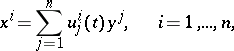(1)

smoothly depending onand transforming a linear system of ordinary differential equations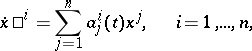(2)

to a system of triangular type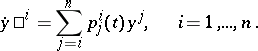(3)

It was introduced by O. Perron . Perron's theorem applies: For any linear system (2) with continuous coefficients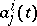, a Perron transformation exists.

A Perron transformation is constructed by means of Gram–Schmidt orthogonalization (for each) of the vector system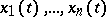, whereis some fundamental system of solutions to (2), where different fundamental systems give, in general, different Perron transformations , . For systems (2) with bounded continuous coefficients, all the Perron transformations are Lyapunov transformations (cf. Lyapunov transformation).

If the matrix-valued function,, is a recurrent function, one can find a recurrent matrix-valued function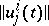,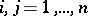, such that (1) is the Perron transformation that reduces (2) to the triangular form (3), where, moreover, the functionis recurrent.